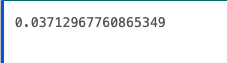# Summary of Java Math.random and import (Calendar)

I'm taking notes of the jammed parts based on the learning site paiza. Math.random() Get double type random numbers in the range of 0.0 to less than 1.0. The return value is double.

``````double number = Math.random();
``````

Output resultWhen you want to get a value from 1 to 100.

``````double number = Math.random() * 100 + 1;
``````

This time, "* 100" is ** how many numbers to put out, the number of ranges ** "+1" is ** how many numbers start, the lower limit value ** Can be entered by specifying the range.

If you want to get the value in the range of 4 to 10 (7 numbers), display as follows.

``````double number = Math.random() * 7 + 4;
``````

If you want the return value to be an integer, cast it.

``````int num = (int)number;
Or
int num = (int)(Math.random());
``````

import (Calendar) A class library that gets the date.

### Description example:

``````import java.util.Calendar;
public class Main {
public static void main(String[] args) {
Calendar calendar = Calendar.getInstance();
int seireki = calendar.get(Calendar.YEAR);

System.out.println(seireki + "Year");
}
}
``````

Get the month

``````int month = calendar.get(Calendar.MONTH);
``````

Get the day

``````int data = calendar.get(Calendar.DATE);
``````

Sites referenced for import [Java] A brief explanation for beginners! Take import from a different angle.

I also wanted to know the class to get time by the way, so the site listed [With sample program] Summary of how to get Java time!